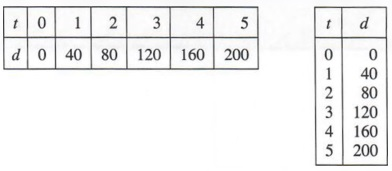# What I learned in Grade 9 Linear Relations

What is a Linear Relation

A linear relation is a pattern that increases or decreases by the same amount every time.What is a T-Chart and How to fill it

When finding the rule for a linear relation, you can put the numbers in a t-chart. A t-chart helps you record your data in one table. This chart helps you find the relationship between x and y. On the left side of the chart is x, and the right is y.How to find the Rule for a Pattern

The first step is to know how much the pattern changes each time. Once you know how much your pattern goes up/down by you put the number next to x. Next you insert 1 instead of x as it is the first number in the pattern. Example: 6(1)=y. You know that 6(1) does not equal 3. The next step is to find how you get from 6to 3. To get from 6 to 3 you subtract 3. Now add -3 to your rule. Your rule now looks like 6x-3=y. To see if this is correct, try it on the next number.How to Plot a Point

On a graph, the horizontal line is the x axis and the vertical line is the y axis. The first number on your coordinates is the x number and the second number is the y. Example: 2,3 the 2 is x and 3 is y. The first number tells you which way you are going to go on the x axis. If the number is positive you go to the right and if the number is negative you go to the left. The second number tells you which way you are going on the y axis. If the number is positive you are going up the y axis and if the number is negative you are going down the y axis. If are coordinate is 2,3 you will go right 2 times, and then up 3 times. Once you have done this, you place your point where the coordinates lead.How to Graph a Linear Relation

To graph a linear relation, put your equation into a t-chart. Once you have a couple pairs of numbers you can insert the points onto the graph. Example, the rule 6x-3=y, the first three numbers are 3,9,15. Now your t-chart has 1,2,3 in the x column, and 3,9,15 in the y column. When you put the two together you get your coordinates. For example, 1,3  2,69 3,15. Once you have these coordinates you can plot them on the graph.How to Graph Vertical and Horizontal Lines

A vertical line goes across the x axis. This means that the formula you are going to use x = a number. For example x=3. A horizontal line goes across the y axis. This means that the formula you are going to use is y = a number. For example y=-3. This will create a horizontal line to the right and left of -23 on the y axis.Vocabulary:

X Axis – The horizontal line on a graph.

Y Axis – The vertical line on a graph.

T-Chart – A chart that has two columns.

Quadrant – A graph that is split in 4 parts.

Origin- The middle of the graph.

Plotting – Where you place your point on a graph.

Linear Pattern – A pattern that increases or decreases by the same amount each time.

Increasing Pattern – A pattern that increases each time.

Decreasing Pattern – A pattern that decreases each time.

Horizontal Line – A line that runs from left to right.

Vertical Line – A line that runs top to bottom.

Something Else I learned

Something else that I learned in this unit is restricting your line to between two points. This is useful because without the formula, you will have a long line running from one side of the graph to the other.